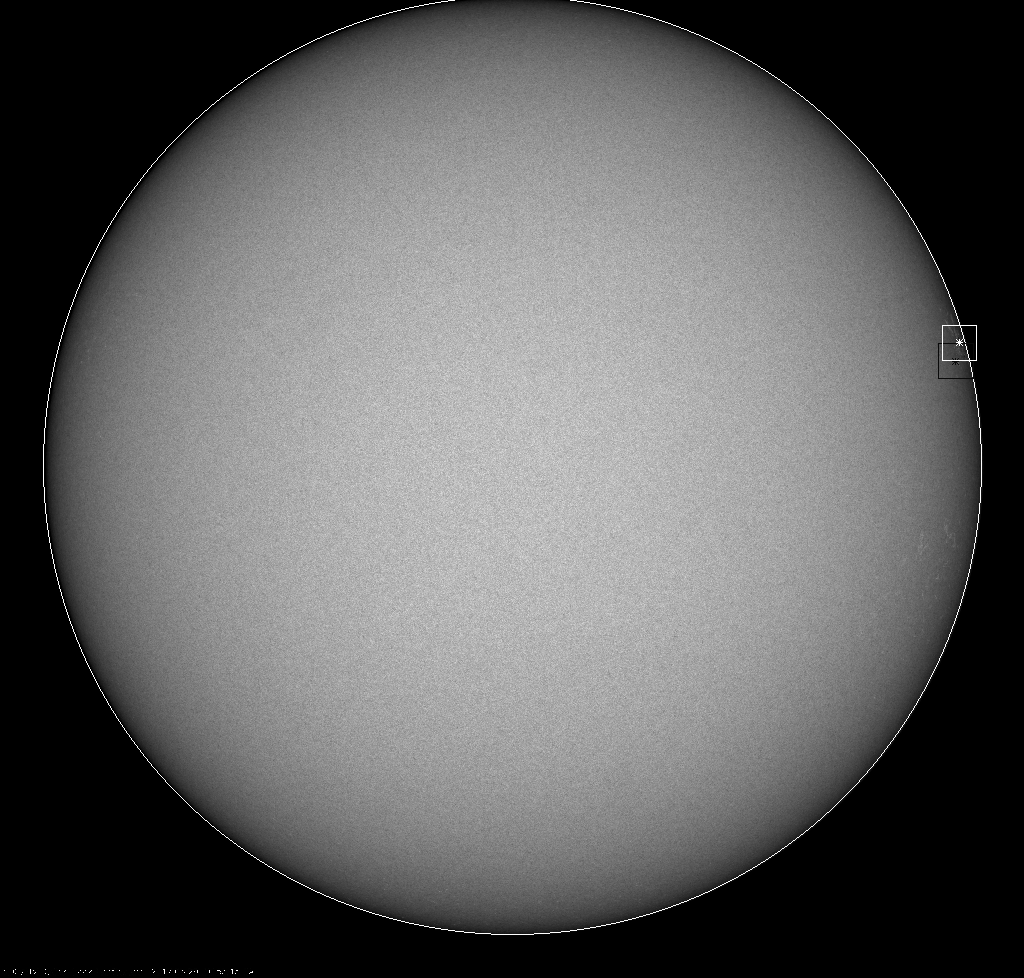; --- NST TiO Fitsheader Corrections Determined from TiO-HMII Co-alignment; Images Used:
; --- bbso_tio_pcosr_20170529_165014.fts and 20170529_165238_4096_HMII.jpg
; --- Generated by "bbso_tio2hmi.pro" on Thu Jun 15 11:04:37 2017
; ---
; --- The Xc and Yc in the TiO Fits header should be corrected by
dXc= 7.55 arcsec, and dYc= 37.45 arcsec
; ---
; --- The corrected coordinates of the center of the FOV in the panels below are (in arcsec)
Xc= 901.55 arcsec and Yc= 247.45 arcsec
; --- Please keep in mind that due to telescope flexture and the seeing the FOV will still move around but the pointing error will be small.
; ---
; --- New TiO Pix size as defined from the co-alignment is (in arcsec)
0.0361 arcsec/pixel
; --- Old (fits header) TiO Pix size is (in arcsec)
0.0342 arcsec/pixel; Correction factor 1.0571x
; ---
; --- Keep in mind that the pix size correction is very tiny and the old pix size is not necessarily incorrect.
; ---
; --- You may use this correction file, in your IDL data processing
; ---
; --- Starting 2016 all TiO images have to be flipped in vertical direction
; --- and then rotated using the fits header parameter CROTA1
; ---
; --- One can also derotate image using the IDL code below ; --- the imput params are ; --- * the current image obs time ("time") ; --- * the reference time ("time1", see explanations in the code) ; --- * current solar Po ; ---
; --- 20170529 Solar Po= -16.288175, deg
; --- 20170529 Solar Radius= 946.73598 arcsec
; --- 20170529 Reference time = 20.949239 hours
; --- Use IDL code below to derotate images
; -------------------------------------------------------------------------------------------------------
PRO DEROTATE_IMG, img, time, time1, direction, MISSING=MISSING, ANGLE=ANGLE, dangle=dangle
;+ ;
;INPUT ; img: image to be derotated
; time: time of the image acquisition in fraction of hours, e.g., 18:20->18.33
; time1: time of the first image in the set, it determines by how much img should be rotated
; it is also the reference time; for example, at approx 21:14UT the orientation of NST FOV ; is aligned along solar N-S and E-W lines.
; By setting time1 to 21.2333 (21:14) any TiO image will be derotated thus to match the HMI orientation.
; HOWEVER, to achieve that you must assign parameter angle = solar_p0! (listed in fits header)
; angle: any additional correction angle, such as solar Po calculated on day of observations
; CCW: if rotation should proceed in CCW direction
;
; all missing values will be filled w/ missing
; output
; dangle is the resulting rotation angle
;
; USAGE example> derotate_img, img, 18.34, 21.2333, '1', missing=999, angle=solar_p0, dangle=dangle
;
; Copyright: May 6 2017 by Vasyl Yurchyshyn, NJIT
;-
IF direction EQ 1 THEN dir=1.
IF direction EQ 2 THEN dir=-1.

IF NOT KEYWORD_SET(angle) THEN angle=0

dt=time-time1
dangle=dt*360./24.
dangle=angle+dangle*dir

img=ROT(img, dangle, /INTERP, MISSING=MISSING)

END
; -------------------------------------------------------------------------------------------------------; ---
; --- The two panel figure shows the resulting coalignment between HMI (left) and TiO (right) images. Both images are overplotted with the same TiO contours.
; ---; ---
; --- The full disk HMI/I image overplotted with 1) the TiO original fitheader center of FOV (black asterisk) and the FOV size (black square) and 2)corrected FOV and the center (white). ; ---